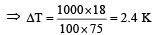Courses

# Test: Thermodynamics 2 - From Past 28 Years Questions

## 30 Questions MCQ Test Chemistry Class 11 | Test: Thermodynamics 2 - From Past 28 Years Questions

Description
This mock test of Test: Thermodynamics 2 - From Past 28 Years Questions for NEET helps you for every NEET entrance exam. This contains 30 Multiple Choice Questions for NEET Test: Thermodynamics 2 - From Past 28 Years Questions (mcq) to study with solutions a complete question bank. The solved questions answers in this Test: Thermodynamics 2 - From Past 28 Years Questions quiz give you a good mix of easy questions and tough questions. NEET students definitely take this Test: Thermodynamics 2 - From Past 28 Years Questions exercise for a better result in the exam. You can find other Test: Thermodynamics 2 - From Past 28 Years Questions extra questions, long questions & short questions for NEET on EduRev as well by searching above.
QUESTION: 1

### If ΔH is the change in enthalpy and ΔE, the change in internal energy accompanying a gaseous reaction, then 

Solution:

As  ΔH = ΔE + ΔngRT if n p < nr; Δ ng = np – nr = – ve.
Hence Δ H < Δ E.

QUESTION: 2

### Equal volumes of molar hydrochloric acid and sulphuric acid are neutralized by dil. NaOH solution and x kcal and y kcal of heat are liberated respectively. Which of the following is true ?

Solution:

1MH2SO4 = 2g eq. of H2SO4.
Hence y = 2x or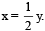QUESTION: 3

### For the reaction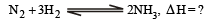Solution:

Δng = 2 – 4 = – 2, ΔH = ΔE – 2RT.

QUESTION: 4

During isothermal expansion of an ideal gas, its

Solution:

During isothermal expansion of ideal gas,
ΔT = 0.   Now H = E + PV
∵ ΔH = ΔE + Δ(PV)
∴ ΔH = ΔE + Δ(nRTT));
Thus if ΔT = 0., ΔH = ΔE i.e., remain unaffected

QUESTION: 5

A chemical reaction will be spontaneous if it is accompanied by a decrease of 

Solution:

ΔG is negative for a spontaneous process.

QUESTION: 6

Consider the following reaction occurring in an automobile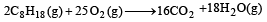the sign of ΔH, ΔS and ΔG would be

Solution:

This is combustion reaction, which is always exothermic hence
ΔH = –ve
As the no. of gaseous molecules are increasing hence entropy increases now ΔG = ΔH - TΔS
For a spontaneous reaction ΔG = -ve
Which is possible in this case as ΔH = –ve and ΔS = +ve.

QUESTION: 7

Standard Gibb’s free energy change for isomerization reaction  cis-2 pentene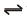trans-2-pentene is – 3.67 kJ/mol at 400 K. If more trans-2 pentene is added to the reaction vessel, then

Solution:

If more tran s-2-pentene is added, then its concentration in right hand side will increase. But in order to maintain the K constant, concentration of cis-2-pentene will also increase. Therefore more cis-2-pentene will be formed.

QUESTION: 8

If enthalpies of formation of C2H4(g) , CO2(g) and H2O(l) at 25°C and 1atm pressure are 52, – 394 and – 286 kJ/mol respectively, the change in ethalpy is equal to 

Solution:

Enthalpy of formation of C2H4 , CO2 and H2O are 52 , – 394 and – 2 86 k J/ mol respectively. (Given) The reaction is
C2H4 + 3O2 → 2CO2 + 2H2O.
change in enthalpy, (ΔH) = ΔHproducts - ΔHreactants
= 2 x (-394) + 2 x (-286) - (52 + 0)
= – 1412 kJ/ mol.

QUESTION: 9

The correct relationship between free energy and equilibrium con stant K of a reaction is 

Solution:

Enthalpy of formation of C2H4 , CO2 and H2O are 52 , – 394 and – 2 86 k J/ mol respectively. (Given) The reaction is
C2H4 + 3O2 → 2CO2 + 2H2O.
change in enthalpy, (ΔH) = ΔHproducts - ΔHreactants
= 2 x (-394) + 2 x (-286) - (52 + 0)
= – 1412 kJ/ mol.

The relation between free energy change and equilibrium constant is given by Nernst equation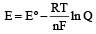At equilibrium,  Ec = 0 and Q = KC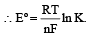................(i)

Again ΔGº = nFEº (ii)

put in (i)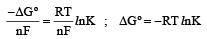QUESTION: 10

According to the third law of thermodynamics which one of the following quantities for a perfectly crystalline solid is zero at absolute zero?

Solution:

Entropy states the randomness or disorderness of the system. At absolute zero, the movement of molecules of the system or randomenss of the system is zero, hence entropy is also zero.

QUESTION: 11

Given the following entropy values (in J K–1 mol–1) at 298 K and 1 atm :H2(g) : 130.6, Cl2(g) : 223.0, HCl (g) : 186.7.The entropy change (in J K–1 mol– 1) for the reaction H2(g) + Cl 2(g)  —→  2 HCl(g) is 

Solution:

Entropy change ΔS =  ΔSproduct - ΔSreactant
= 2 (186.7) – (223 + 130.6)
= 373.4 – 353.6
= 19.8 JK–1 mol–1

QUESTION: 12

Hydrogen has an ionisation energy of 1311 kJ mol–1 and for chlorine it is 1256 kJ mol–1.Hydrogen forms H+ (aq) ions but chlorine does not form Cl+ (aq) ions because 

Solution:

Hydration energy of Cl+ is very less than H+ hence it doesn’t form Cl+ (aq) ion.

QUESTION: 13

Given that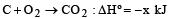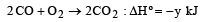the enthalpy of formation of carbon monoxide will be 

Solution:

Given C + O2 = CO2 , ΔHº =-x kJ    ....(1)
2CO2 = 2CO +O2 ΔHº = + y kJ  … (2)
or CO2 = CO+ 1/2O2, ΔHº =+ y/2 kJ ...(3)
From eq. no. (1) and (3)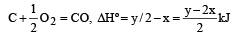QUESTION: 14

Identify the correct statement regarding entropy: 

Solution:

We know from the third law of thermodynamics, the entropy of a perfectly crystalline substance at absolute zero temperature is taken to be zero.

QUESTION: 15

One mole of an ideal gas at 300 K is expanded isothermally from an initial volume of 1 litre to 10 litres. The ΔE for this process is (R =2 cal. mol–1 K–1)

Solution:

For an isother mal process ΔE  = 0

QUESTION: 16

For a cyclic process, which of the following is not true? 

Solution:

For a cyclic process

ΔE = 0, ΔH = 0 & ΔG = 0 .

As all depend upon final state and initial state,w doesn’t depend on path followed.

QUESTION: 17

Adiabatice xpansions of an ideal gas is accompanied by 

Solution:

ΔE = ΔQ–W
⇒  ΔE = –W
The negative sign shows decrease in Internal energy, which is equal to the work done on the system by the surroundings.

QUESTION: 18

For a reaction in which all reactants and products are liquids, which one of the following equations is most applicable ? 

Solution:

As all reactant and product are liquid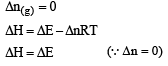QUESTION: 19

The entropy change in the fusion of one mole of a solid melting at 27ºC (Latent heat of fusion, 2930 J mol–1) is : 

Solution: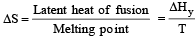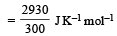= 9.77 J K–1 mol–1

QUESTION: 20

The factor of ΔG values is important in metallurgy. The ΔG values for the following reactions at 800ºC are given as :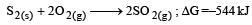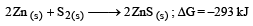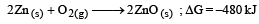Then ΔG for the reaction :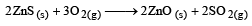will be :

Solution:

For the reaction 2ZnS → 2Zn + S2 ; ΔG1º = 293 kJ  ..........(1)
2Zn + O2 → ZnO ; ΔG2º = –480 kJ  ..........(2)
S2 + 2O2 → 2 SO2 ; ΔG3º = –544 kJ  .........(3)
ΔGº for the reaction 2ZnS + 3O2 → 2ZnO + 2SO2 can be obtained by adding eqn. (1), (2) and (3)
⇒ ΔGº = 293 – 480 – 544 = – 731 kJ

QUESTION: 21

The values of heat of formation of SO2 and SO3 are –298.2 kJ and –98.2 kJ. The heat of formation of the reaction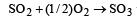will be 

Solution: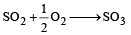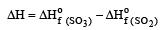= –98.2 + 298.2 = 200 kJ/Mole

QUESTION: 22

For a hypothetical reaction A → B , the activation energies for forward and backward reactions are 19 kJ/mole and 9 kJ/mole respectively. The heat of reaction is 

Solution:

Solution:-

As we know that, for a reaction,

ΔH=Ea​ for forward reaction −Ea​ for backward reaction.

Given that:-

Ea​ for forward reaction =19kJ

Ea​ for backward reaction =9kJ

∴ΔH=19−9=10kJ

QUESTION: 23

Enthalpy of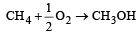is negative. If enthalpy of combustion of CH4 and CH3OH are x and y respectively, then which relation is correct 

Solution: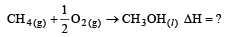∴ ΔH = [(ΔH of combustion of CH3OH) = (ΔH of combustion of CH4)]
= [(–y) – (–x)]  = –[–y+x] =  x – y
Given ΔH = –ve
∴ x – y < 0 hence x < y

QUESTION: 24

When 1 mol of a gas is heated at constant volume, temperature is raised from 298 to 308 K. If heat supplied to the gas is 500 J, then which statement is correct ? 

Solution:

As volume is constant hence work done in this proces is zero hence heat supplied is equal to change in internal energy.

QUESTION: 25

What is the enth alpy chan ge for, 2H2O2(l) → 2 H2O(l) + O2(g) if h eat of formation of H2O2 (l) and H2O (l) are –188 and – 286 kJ/mol respectively? 

Solution: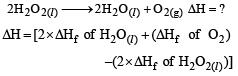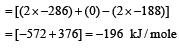QUESTION: 26

In a closed insulated container, a liquid is stirred with a paddle to increase the temperature, which of the following is true? 

Solution:

Internal energy is dependent upon temperature and according to first law of thermodynamics total energy of an isolated system remains same, i.e., in a system of constant mass, energy can neither be created nor destroyed by any physical or chemical change but can be transformed from one form to another
ΔE = q + w For closed insulated container, q = 0, so,
ΔE = +w , as wor k is done by the system

QUESTION: 27

2 mole of an ideal gas at 27ºC temperature is expanded reversibly from 2 lit to 20 lit. Find the entropy change (R = 2 cal/mol K) 

Solution:

For isothermal reversible expansion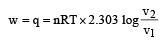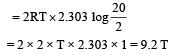Entropy change,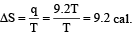QUESTION: 28

Heat of combustion ΔHº for C (s), H2(g) and CH4(g) are –94, –68 and –213 kcal/mol, then ΔHº for C(s) + 2H2(g) → CH4(g) is 

Solution: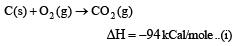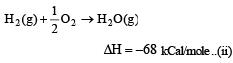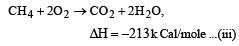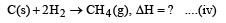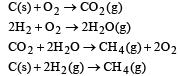QUESTION: 29

Unit of entropy is 

Solution: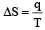q —→ required heat per mole
T —→ constant absolute temperature
Unit of entropy is JK–1 mol–1

QUESTION: 30

The molar heat capacity of water at constant pressure is 75 JK–1 mol–1. When 1kJ of heat is supplied to 100 g of water, which is free to expand, the increase in temperature of water is 

Solution:

Given Cp = 75 JK–1 mol–1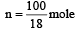Q = 1000J         ΔT = ?

Q = nCpΔT